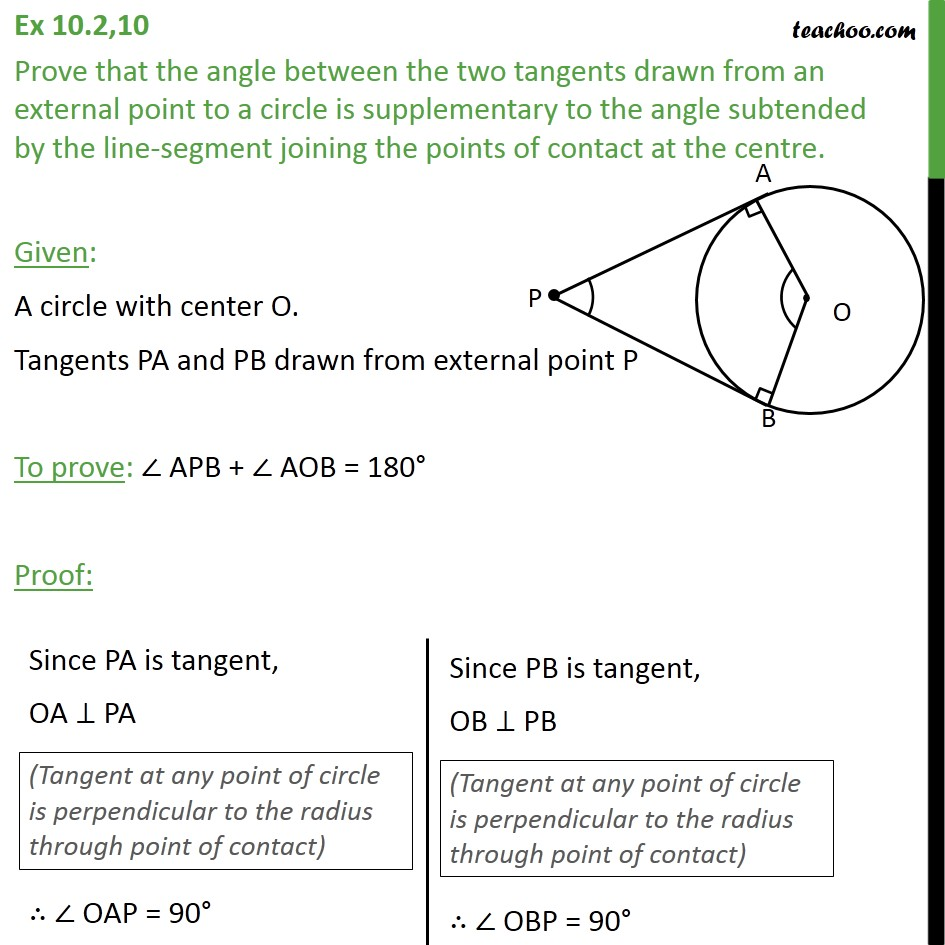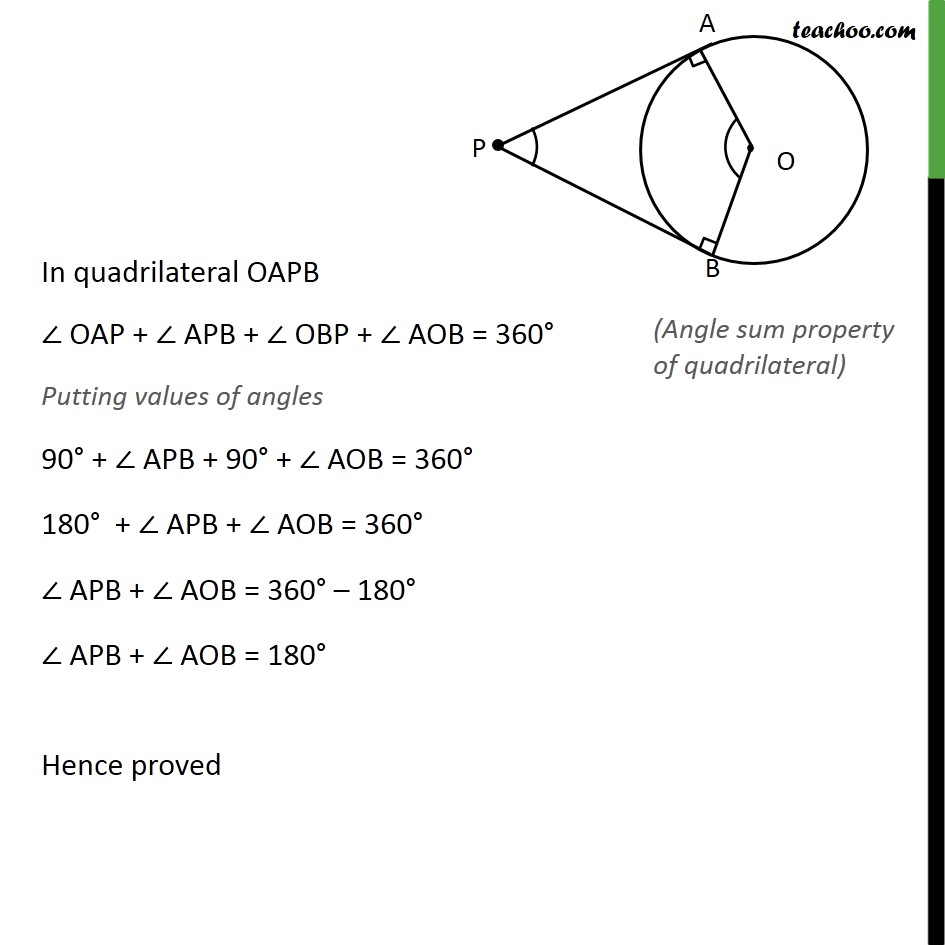Ex 10.2

Chapter 10 Class 10 Circles
Serial order wiseLearn in your speed, with individual attention - Teachoo Maths 1-on-1 Class

### Transcript

Ex 10.2,10 Prove that the angle between the two tangents drawn from an external point to a circle is supplementary to the angle subtended by the line-segment joining the points of contact at the centre. Given: A circle with center O. Tangents PA and PB drawn from external point P To prove: APB + AOB = 180 Proof: In quadrilateral OAPB OAP + APB + OBP + AOB = 360 Putting values of angles 90 + APB + 90 + AOB = 360 180 + APB + AOB = 360 APB + AOB = 360 180 APB + AOB = 180 Hence proved